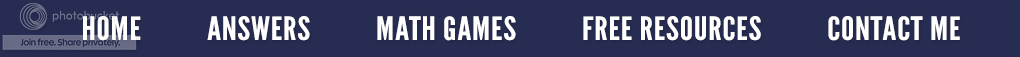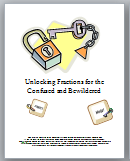### The Power of Math Tricks

Math tricks will never make you a great mathematician, but in the eyes of some, you can be a fantastic math-a-magician. My college students love it when I show them a trick they can then take home to amaze and impress their peers, parents, children or the best yet - their spouses.

Remember when I demonstrated how to easily multiply by 11 in the post The Eleventh Hour?  Or how about the trick of multiplying by 12 in Quick Times?   Here is a new one I recently showed my students.

First, let's look at a problem where a number is divided by the decimal .25

The above example is really 9 x 4 which is 36, but why is this true?  Hopefully your students know that .25 is equivalent to 1/4; so this problem can be reworded as  9 divided by 1/4.  As seen below, when dividing a whole number by a fraction, we find the reciprocal of 1/4 and then multiply which gives us the answer of 36.

Based on the sample above, anytime a problem requires dividing by .25, simply multiply by four to get the correct answer.  Try these without using a calculator or paper and pencil.

﻿
﻿
Instead of using the reciprocal to divide fractions, I teach my students that this is the "cross" method. Simply look at the original problem and cross multiply as seen in the illustration below.
﻿Fractions for the Confused and Bewildered

First multiply the bottom right denominator with the top left numerator. (4 x 9)  Next multiply the bottom left denominator with the top right numerator, (1 x 1) and you get an answer of 36.  When doing the fractions this way, there is no confusion on the students' part about which fraction to invert. If you would like a more details on how to divide fractions this way, go to the post entitled: Don't Flip!

If you are interested in other alternative ways to teach the four operations of fractions, you can check out the resource on your right.

By the way, the answers to the above problems are  a) 24    b) 80    c) 72    d) 380.    How did you do?